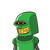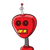# fill in the blanks:i, if a number is added to two-fifth of itself,the value so obtained is 455. the number is____________​

fill in the blanks:i, if a number is added to two-fifth of itself,the value so obtained is 455. the number is____________​

### 2 thoughts on “fill in the blanks:i, if a number is added to two-fifth of itself,the value so obtained is 455. the number is____________​”

1.Let the number be x

Then,

⇔x+25x=455⇔75x=455⇔x=(455×57)⇔x=325

2.Step-by-step explanation: